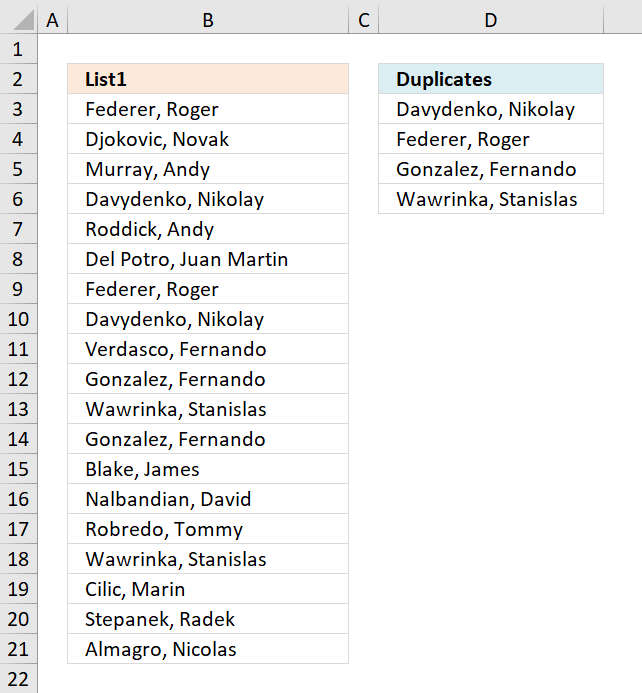Author: Oscar Cronquist Article last updated on November 22, 2018The following array formula extracts duplicate values sorted from A to Z from cell range B3:B21.

Excel array formula in C2:

=INDEX(\$A\$2:\$A\$20, MATCH(MIN(IF(COUNTIF(\$A\$2:\$A\$20, \$A\$2:\$A\$20)*IF(COUNTIF(C1:\$C\$1, \$A\$2:\$A\$20)=1, 0, 1)>1, COUNTIF(\$A\$2:\$A\$20, "<"&\$A\$2:\$A\$20), "")), IF(COUNTIF(\$A\$2:\$A\$20, \$A\$2:\$A\$20)>1, COUNTIF(\$A\$2:\$A\$20, "<"&\$A\$2:\$A\$20), ""), 0))

#### How to enter an array formula

1. Copy above formula
2. Double press with left mouse button on cell D3
3. Paste formula to cell D3
4. Press and hold CTRL + SHIFT simultaneously
5. Press Enter once
6. Release all keys

The formula now looks like this: {=arrayformula}

Don't enter the curly brackets yourself, they appear automatically.

### Explaining formula in cell C2

#### Step 1 - Count values

The COUNTIF fucntion counts values based on a condition or criteria, this way we can identify which values are duplicates and which are not.

COUNTIF(\$B\$3:\$B\$21,\$B\$3:\$B\$21)

becomes

COUNTIF({"Federer, Roger "; "Djokovic, Novak "; "Murray, Andy "; ... ; "Almagro, Nicolas "},{"Federer, Roger "; "Djokovic, Novak "; "Murray, Andy "; ... ; "Almagro, Nicolas "})

and returns

{2;1;1;2;1;1;2;2;1;2;2;2;1;1;1;2;1;1;1}.

#### Step 2 - Display first instance of each duplicate

The following COUNTIF function makes sure that the output list only contains unique values. The first argument contains a cell reference that expands as the formula is copied to cells below.

COUNTIF(D2:\$D\$2,\$B\$3:\$B\$21)<>1

becomes

COUNTIF("Duplicates",{"Federer, Roger "; "Djokovic, Novak "; "Murray, Andy "; ... ; "Almagro, Nicolas "})<>1

becomes

{0;0;0;0;0;0;0;0; 0;0;0;0;0;0; 0;0;0;0;0}<>1

and returns

{TRUE; TRUE; TRUE; TRUE; TRUE; TRUE; TRUE; TRUE; TRUE; TRUE; TRUE; TRUE; TRUE; TRUE; TRUE; TRUE; TRUE; TRUE; TRUE}.

#### Step 3 - Multiply arrays

Both values must return TRUE.

COUNTIF(\$B\$3:\$B\$21,\$B\$3:\$B\$21)*(COUNTIF(D2:\$D\$2,\$B\$3:\$B\$21)

becomes

{2;1;1;2;1;1;2;2;1;2;2;2;1;1;1;2;1;1;1}*{TRUE; TRUE; TRUE; TRUE; TRUE; TRUE; TRUE; TRUE; TRUE; TRUE; TRUE; TRUE; TRUE; TRUE; TRUE; TRUE; TRUE; TRUE; TRUE}

and returns

{2; 1; 1; 2; 1; 1; 2; 2; 1; 2; 2; 2; 1; 1; 1; 2; 1; 1; 1}.

 Boolean Boolean Multiply FALSE FALSE 0 FALSE TRUE 0 TRUE TRUE 1

#### Step 4 - Replace TRUE with a number representing the order if list were sorted

The IF function returns a value based on a logical expression, if TRUE the second argument is returned, if FALSE the third argument.

IF(COUNTIF(\$B\$3:\$B\$21,\$B\$3:\$B\$21)*(COUNTIF(D2:\$D\$2,\$B\$3:\$B\$21)<>1)>1,COUNTIF(\$B\$3:\$B\$21,"<"&\$B\$3:\$B\$21),"")

becomes

IF({2; 1; 1; 2; 1; 1; 2; 2; 1; 2; 2; 2; 1; 1; 1; 2; 1; 1; 1}>1,COUNTIF(\$B\$3:\$B\$21,"<"&\$B\$3:\$B\$21),"")

becomes

IF({TRUE; FALSE; FALSE; TRUE; FALSE; FALSE; TRUE; TRUE; FALSE; TRUE; TRUE; TRUE; FALSE; FALSE; FALSE; TRUE; FALSE; FALSE; FALSE},COUNTIF(\$B\$3:\$B\$21,"<"&\$B\$3:\$B\$21),"")

becomes

IF({TRUE; FALSE; FALSE; TRUE; FALSE; FALSE; TRUE; TRUE; FALSE; TRUE; TRUE; TRUE; FALSE; FALSE; FALSE; TRUE; FALSE; FALSE; FALSE},{7;6;11;3;14;5;7;3;16;9;17;9;1;12;13;17;2;15;0},"")

and returns

{7;"";"";3;"";"";7;3;"";9;17;9;"";"";"";17;"";"";""}.

#### Step 5 - Get smallest value in array

The MIN function returns the samllest value in a cell range or array.

MIN(IF(COUNTIF(\$A\$2:\$A\$20, \$A\$2:\$A\$20)*IF(COUNTIF(C1:\$C\$1, \$A\$2:\$A\$20)=1, 0, 1)>1, COUNTIF(\$A\$2:\$A\$20, "<"&\$A\$2:\$A\$20), ""))

becomes

MIN({7;"";"";3;"";"";7;3;"";9;17;9;"";"";"";17;"";"";""})

and returns 3.

#### Step 6 - Replace TRUE in array with a number representing the order if list were sorted

IF(COUNTIF(\$B\$3:\$B\$21,\$B\$3:\$B\$21)>1,COUNTIF(\$B\$3:\$B\$21,"<"&\$B\$3:\$B\$21),"")

becomes

IF({TRUE; FALSE; FALSE; TRUE; FALSE; FALSE; TRUE; TRUE; FALSE; TRUE; TRUE; TRUE; FALSE; FALSE; FALSE; TRUE; FALSE; FALSE; FALSE}, COUNTIF(\$B\$3:\$B\$21,"<"&\$B\$3:\$B\$21),"")

becomes

IF({TRUE; FALSE; FALSE; TRUE; FALSE; FALSE; TRUE; TRUE; FALSE; TRUE; TRUE; TRUE; FALSE; FALSE; FALSE; TRUE; FALSE; FALSE; FALSE}, {7;6;11;3;14;5;7;3;16;9;17;9;1;12;13;17;2;15;0},"")

and returns {7;"";"";3;"";"";7;3;"";9;17;9;"";"";"";17;"";"";""}.

#### Step 7 - Return relative position

MATCH(MIN(IF(COUNTIF(\$B\$3:\$B\$21,\$B\$3:\$B\$21)*(COUNTIF(D2:\$D\$2,\$B\$3:\$B\$21)<>1)>1,COUNTIF(\$B\$3:\$B\$21,"<"&\$B\$3:\$B\$21),"")),IF(COUNTIF(\$B\$3:\$B\$21,\$B\$3:\$B\$21)>1,COUNTIF(\$B\$3:\$B\$21,"<"&\$B\$3:\$B\$21),""),0)

becomes

MATCH(3, {7;"";"";3;"";"";7;3;"";9;17;9;"";"";"";17;"";"";""},0)

and returns 4.

#### Step 8 - Return value

The INDEX function returns a value from a cell range based on a row and column number. The cell range is a single column so the column number is not neccessary.

INDEX(\$A\$2:\$A\$20, MATCH(MIN(IF(COUNTIF(\$A\$2:\$A\$20, \$A\$2:\$A\$20)*IF(COUNTIF(C1:\$C\$1, \$A\$2:\$A\$20)=1, 0, 1)>1, COUNTIF(\$A\$2:\$A\$20, "<"&\$A\$2:\$A\$20), "")), IF(COUNTIF(\$A\$2:\$A\$20, \$A\$2:\$A\$20)>1, COUNTIF(\$A\$2:\$A\$20, "<"&\$A\$2:\$A\$20), ""), 0))

becomes

INDEX(\$A\$2:\$A\$20, 4)

and returns "Davydenko, Nikolay " in cell D3.

### Get excel sample file for this tutorial

how-to-extract-a-list-of-duplicates-sorted-a-to-z-from-a-column.xlsx